• 转化上下文文法为push down automata，输入文件识别；第一行为文件数，之后为文法，想转化为pda，然后再判断识别
• 正规文法的特性 1.所有长度有限的语言都是正规的。 2.用正规文法当然能产生无限长串，其中周期...我们把上下文无关文法中的正规文法去掉，剩下的那部分我们叫做真正的上下文无关文法。 自嵌入特性是区分真正的上下文无
正规文法的特性
1.所有长度有限的语言都是正规的。
2.用正规文法当然能产生无限长串，其中周期重复部分的长度不大于非终止符的长度。
举个例子

在此规则之下，能生成句子
其中周期重复部分为ab，这个例子的非终止符的元素个数为2，故满足2不大于2.

自嵌入特性
我们把上下文无关文法中的正规文法去掉，剩下的那部分我们叫做真正的上下文无关文法。
自嵌入特性是区分真正的上下文无关文法与正规文法的判定标准。
即一个真正的上下文无关文法一定具有自嵌入特性，正规文法具有非自嵌入特性。亦即非自嵌入的上下文无关文法是正规文法，上下文无关文法就蜕化了。

什么是自嵌入特性？
自嵌入顾名思义，就是能够自己嵌入自己：

当然必须保证v和x不能是空串。

uvwxy定理
这是一个用以判定上下文无关文法和正规文法的条件。
就是说，当这个文法满足自嵌入条件，表示出来就是

那么，可以得到

其中当v和x的重复次数相同且为非空串时，则这个文法肯定就是真正的上下文无关文法。因为这种周期形式是正规文法所不具有的。比如这种

就是必须要用真正的上下文无关文法。

下面介绍上下文无关文法的等价文法。
不同的上下文无关文法，它们生成的语言有可能是等价的，这样就涉及到一个最优文法的问题。那么最优是什么？最有就是高效、没有冗余和浪费。

粗略说有2种冗余，而且只有非终止符能够冗余。终止符总是有用的啦。
其一是浪费环节。
浪费环节是说由单个非终止符传递到了单个的非终止符，比如

这种形式意味着B是多此一举的，还不如直接A到C呢。

其二是无用环节。
细分又有2类。通俗说
1.无尾的无用非终止符
所谓无尾，就是咱用了这个终止符，话根本都没法结束。有头无尾。
A根本没法最后变成常量（终止符）。
不存在x使得

2.无头的无用非终止符
所谓无头，就是这句话根本就不可能从这个非终止符开始。有尾无头。
从起始符开始根本找不到含有A的句子。
从起始符S开始，不存在

首先介绍如何消去浪费环节。
消去浪费环节包括2个部分。
其一消去。
其二修正。

消去过程
这个过程用递归算法来实现。
就是要求出所有非终止符各自对应能到达的所有非终止符集合。

举个例子就好了

现在消去浪费环节，先考虑S，
发现S能在一步之内的非终止符只有A
记
在K1(S)集合中再次出发，在一步之内能走到的新非终止符只有B
记
再往后没有新的非终止符了。
同理考察A，A在一步之内能到达的非终止符只有B了，记
再考察B，B根本就没有能在一步之内能到达的新终止符。

于是这对应的3个集合就求完了

修正过程
修正过程就按照上面的消去过程打补丁就好了，因为要消去一堆连接，旧桥拆了总得重新修吧。还是上面那个例子，先把之前的生成关系画出来，如图1黑线所示。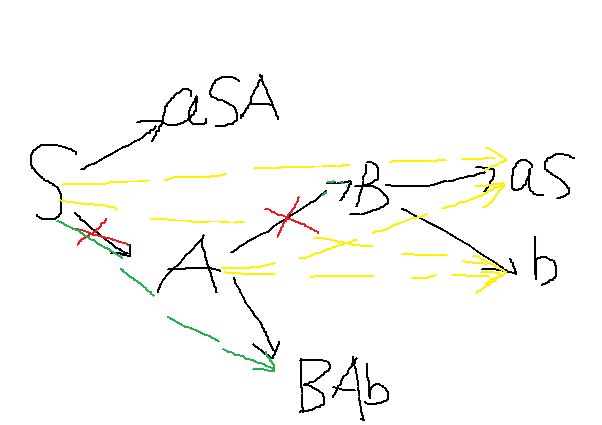图1
现在考虑A，根据集合，我们要去掉A到B的连接
那么就得补上S到b、A到b、S到aS、A到aS，黄线代表。

然后再考虑S，要去掉S到A
那么就得补上S到BAb，绿线代表。

注意：现在虽然看起来文法规则比以前更麻烦了，但是，实际生成句子的过程却变得简单了许多，所以简化没简化还是要看疗效。

下面介绍消去无用的终止符
消去无用终止符的思路就是，找出所有有用的非终止符，那么剩下的自然就是没有用的了。

消去无尾非终止符
找出所有的有尾非终止符集合，定义为J(G)
方法是

这个表达式很清晰，还是举个例子，反而绕口，但还是举吧

这个表达式其实是一步步找到那些最终的到达终止符的非终止符。
首先J0(G)为空集。则J1(G)为所有能一步走到以终止符为尾的非终止符，即A
继续，J2(G)是J1(G)并上所有能在一步之内到以J1(G)或者其他终止符为尾的非终止符。
好绕口。。。其实就是{S,A}
再往下就找不到了。不过现在忽然发现，B呢？怎么没看到B的影子。
这就说明B就是无尾的无用非终止符。

去掉所有跟B有过关系的生成关系就好，现在是新的生成关系

再说说消去无头非终止符
也是只要找出所有有头的非终止符就好，剩下自然就无头了
用表达式表示是这样的

这个表达式的意义其实就是从起始符，回溯一步步展开，希望能找到句子的头部。

还是举个例子

展开起始符S，发现S能到aAA，因此R1(S)={S,A}
A继续展开，发现A能到aCA，因此R2(S)={S,A,C}
搞定，又发现原来B是无头的非终止符，赶紧删掉与它有关系的所有表达关系就好了，不再赘述。

上下文无关文法的2中标准型
1.C（Chomsky）标准型

举一个例子立即就知道怎么把随便一个上下文无关文本变成C标准型了：

2.G（Greibach）标准型

这个严格的转化方法有点畸形，就暂时不展开了。简单情况用凑的就好了。

欢迎参与讨论并关注本博客和微博以及知乎个人主页，后续内容继续更新哦~

转载请您尊重作者的劳动，完整保留上述文字以及本文链接，谢谢您的支持！

展开全文递归算法
• I . 代数表达式 语法 II . 代数表达式 语法 示例 III . 设计 上下文无关语法 IV . 确定性有限自动机 DFA 转为 上下文无关语法
文章目录I . 代数表达式 语法II . 代数表达式 语法 示例III . 设计 上下文无关语法IV . 确定性有限自动机 DFA 转为 上下文无关语法

I . 代数表达式 语法

1 . 代数表达式 语法 : $G4 = ( V , A , R , Expression )$ 是代数表达式语法 ;

① 终端字符集 : $A = \{ a , + , \times , () \}$ ;
② 变量集 :  $V = \{ Expression , Term , Factor \}$ ;

$Expression$ 是表达式 ;
$Term$ 是项 ;
$Factor$ 是因子 ;

2 . $Expression$ 表达式 规则 :
$Expression \to Expression + Term \quad | \quad Term$
$Expression$ ( 表达式 ) 可以通过 $Expression + Term \quad | \quad Term$ 代替 ;

3 . $Term$ 项 规则 :
$Term \to Term \times Factor \quad | \quad Factor$
$Term$ 项 可以通过 $Term \times Factor \quad | \quad Factor$ 代替 ;

4 . $Factor$ 因子 规则 :
$Factor \to Expression \quad | \quad a$
$Factor$ 因子 可以通过 $Expression \quad | \quad a$ 代替 ;

II . 代数表达式 语法 示例

为字符串 $(a + a) \times a$ 构建 语法分析树 ;

1 . 起始状态 : 语法的起始状态是 $Expression$ , 根据 $Expression \to Expression + Term \quad | \quad Term$ 规则 , $Expression$ 可以使用 $Term$ 替换 , 直接从起始状态 , 使用 $Term$ 替换  $Expression$ ;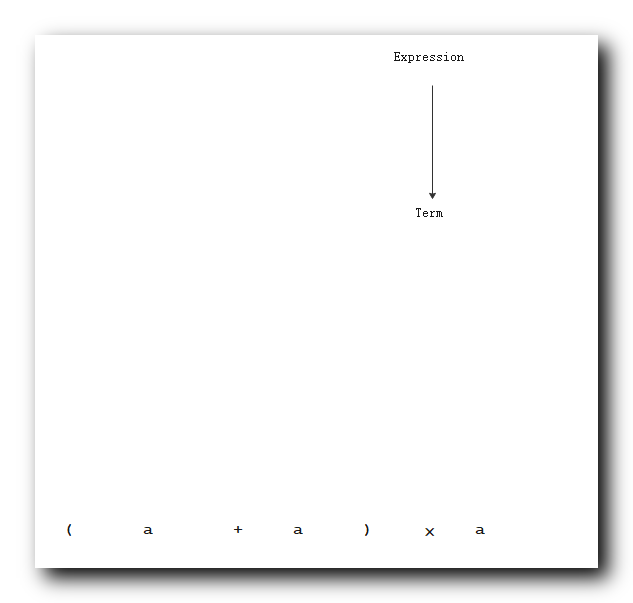2 . $(a + a) \times a$ 字符串的语法分析树 :

符号 : 其中有 $\times$ 乘号 ;
语法分析树 : $(a + a) \times a$ 中间有 $\times$ , 带有 $\times$ 乘号的替换规则为 $Term \to Term \times Factor | Factor$ , 显然该项很显然是一个 $Term$ 项 ;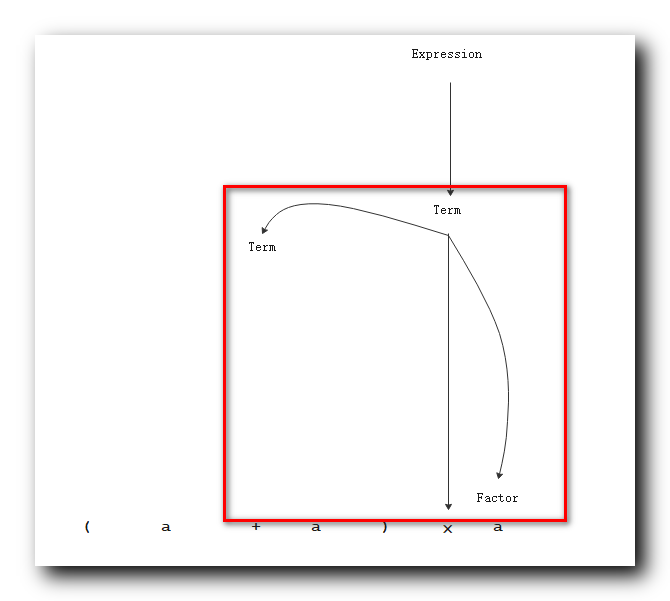3 . 根据上述 $Term \to Term \times Factor$ 可知 , $Term$ 是由 $Term \times Factor$ 进行替换的 , 左侧的 $(a + a)$ 是一个 $Term$ , 右侧的 $a$ 是一个 $Factor$ ;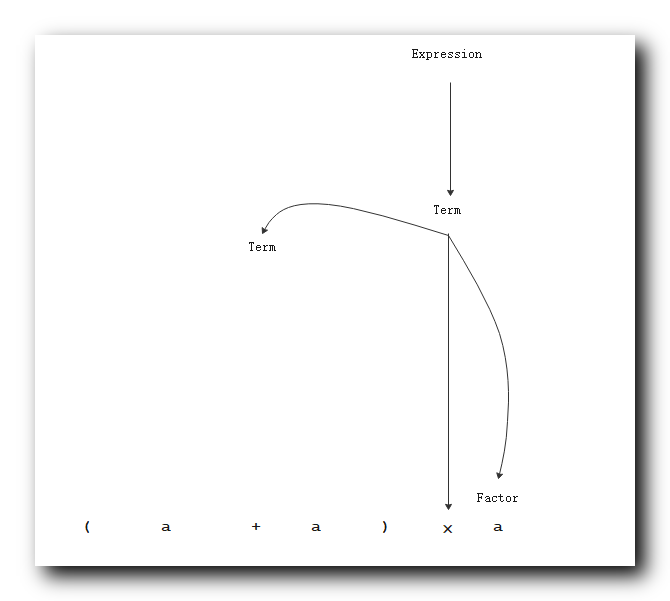4 . 根据  $Factor \to Expression | a$ 规则 , 右侧的 $Factor$ 直接使用 $a$ 进行替代 , 可得如下语法分析树 :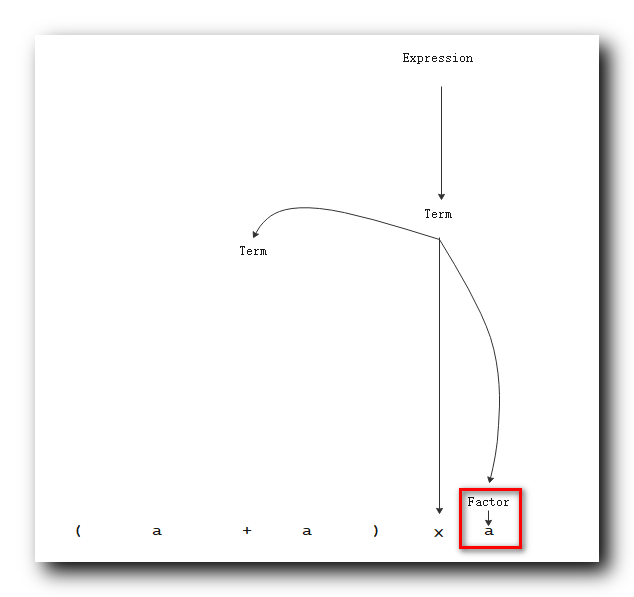5 . 想办法将左侧的 $Term$ 替换成 $(a + a)$ :

加法只能是 $Expression$ , 先替换成 $Expression$ ;

6 . 这里先使用 $Factor$ 替换 $Term$ : 使用规则 $Term \to Term \times Factor | Factor$ ;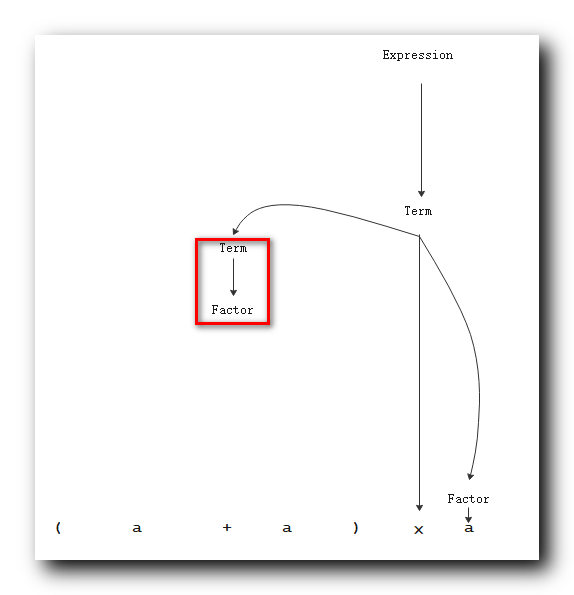7 . 在使用 $Expression$ 替换 $Factor$ : 使用规则 $Factor \to Expression | a$ ;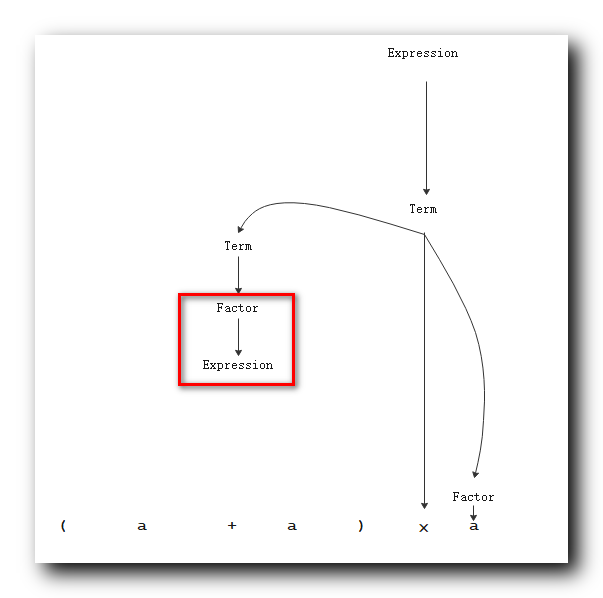8 . 使用 $Expression + Term$ 替换 $Expression$ : 使用规则 $Expression \to Expression + Term | Term$ ;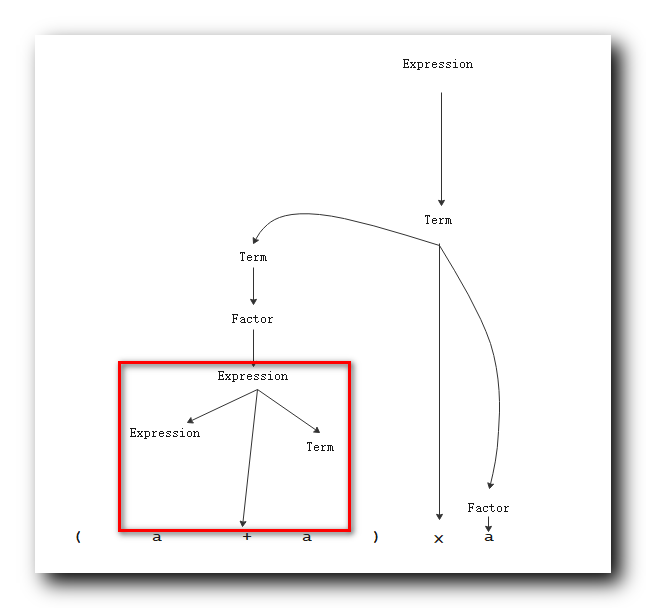9 . 使用 $Term$ 替换 左侧的 $Expression$ : 使用规则 $Expression \to Expression + Term | Term$ ;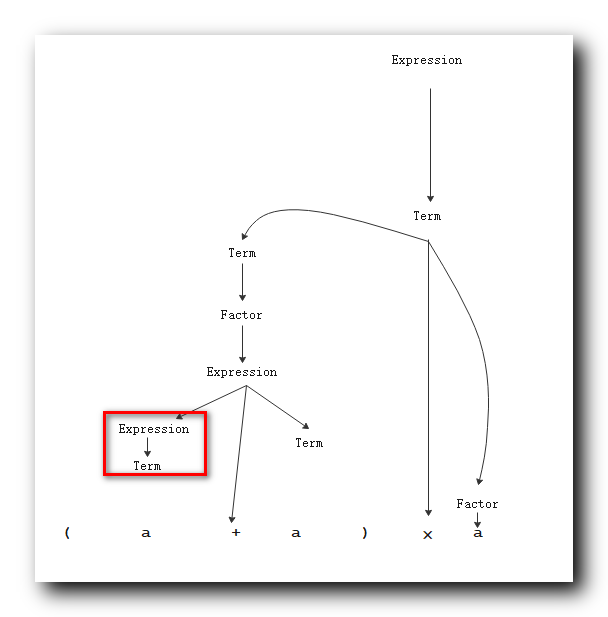10 . 使用 $Factor$ 替换左侧的 $Term$ : 使用规则 $Term \to Term \times Factor | Factor$ ;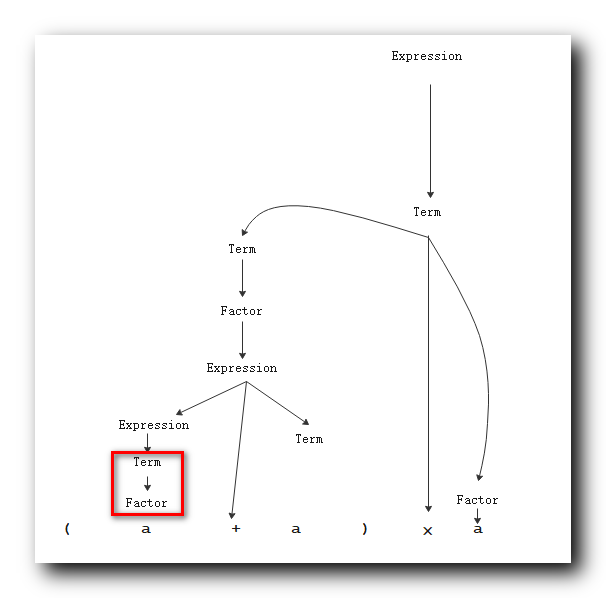11 . 使用 $a$ 替换左侧的 $Factor$ : 使用规则 $Factor \to Expression | a$ ;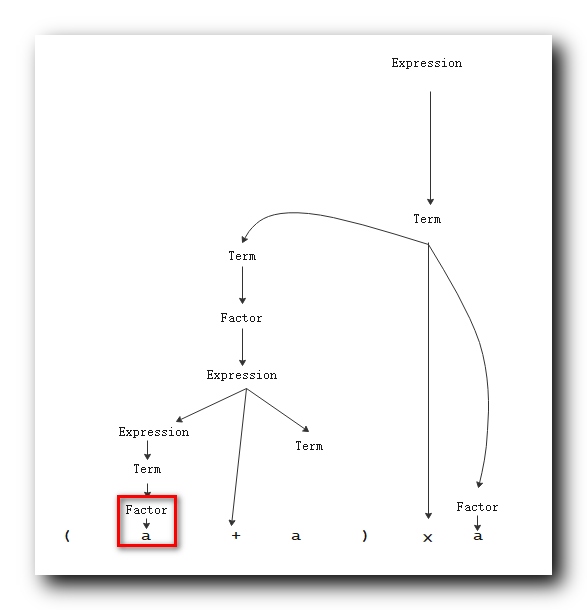12 . 使用 $Factor$ 替换右侧的 $Term$ : 使用规则 $Term \to Term \times Factor | Factor$ ;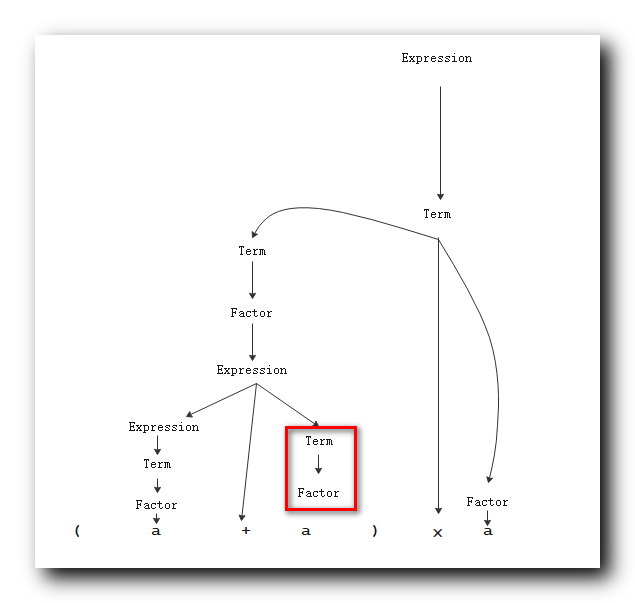13 . 使用 $a$ 替换右侧的 $Factor$ : 使用规则 $Factor \to Expression | a$ ;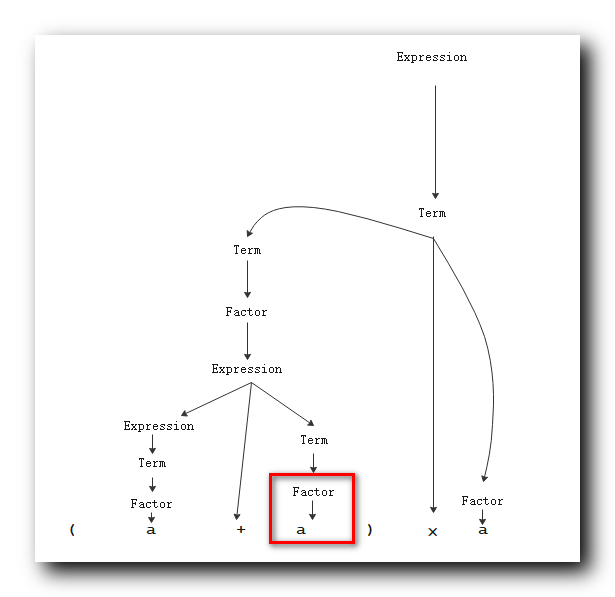最终的 语法分析树为 :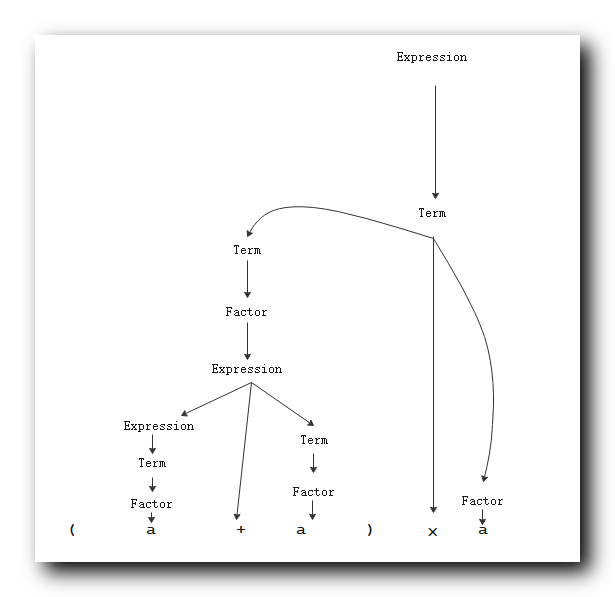此时可以得到语法分析树 ; 该语法分析树是一个代数表达式 ; 将该语法分析树写出 , 即可理解 上下文无关 语法 ;
代数表达式就是上下文无关的语法 ;

III . 设计 上下文无关语法

给定语言 , 设计上下文无关语法 , 使用该语法可以生成该语言 ;

上下文无关语法 设计技巧 : 将复杂的语言分解 , 化整为零 ,  针对每个部分设计上下文无关的语法 ,  最终将这些语法合并在一起 ;

上下文无关语法 与 自动机 : 如果给定的语言是正则语言 , 是由正则表达式表达的 , 能够找到一个自动机可以识别该语言 , 首先将语言转换成自动机 , 将自动机转化为上下文无关的语法 ;

IV . 确定性有限自动机 DFA 转为 上下文无关语法

1 . 确定性有限自动机 ( DFA ) 转为 上下文无关语法 ( CFG ) : 将 确定性有限自动机 ( DFA ) 的指令 , 转为 对应的 上下文无关语法 ( CFG ) 规则 :
$\delta ( q_i, a ) = q_j \Rightarrow R_i \to aR_j$
$\delta ( q_i, a ) = q_j$ 表示 $q_i$ 状态下 , 读取字符 $a$ , 跳转到 $q_j$ 状态 ;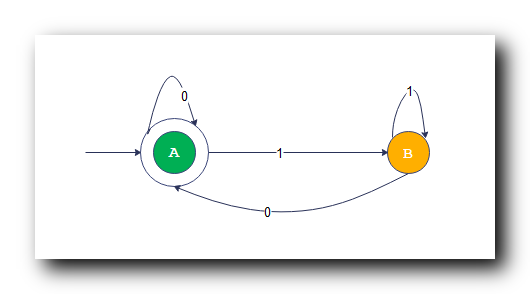2 . 自动机的 状态跳转 转换成 规则 示例 : 上图中的 确定性有限自动机 , 开始状态 $A$ 读取 $1$ 字符 转化成 $B$ 状态 , 表示成规则就是
$R_A \to 1R_B$

3 . 自动机的状态 $A$ 读取 字符 $a$ 转换成另一个状态 $B$ , 都可以转换成对应的规则 $R_A \to aR_B$ ;

4 . 计算能力对比 : 上下文无关语法 的计算能力 要大于等于 自动机的计算能力 ;


展开全文计算理论 代数表达式 确定性有限自动机
• I . 上下文无关语法 设计 示例 II . 上下文无关语法 的歧义性 III . Chomsky 范式 IV . 上下文无关语法 转为 Chomsky 范式 V . 上下文无关语法 转为 Chomsky 范式 示例
文章目录一、上下文无关语法 设计 示例二、上下文无关语法 的歧义性三、Chomsky 范式四、上下文无关语法 转为 Chomsky 范式五、上下文无关语法 转为 Chomsky 范式 示例

一、上下文无关语法 设计 示例

1 . 上下文无关语法 设计要求 : 设计一个语法 , 使用该语法生成语言 $w$ , 该 $w$ 语言的字符串的开始和结尾的字符是相同的 ;

2 . 设计方法 : 非确定性优先自动机 ( NFA ) 识别某语言 , 将 NFA 转为 确定性优先自动机 ( DFA ) , 然后将 DFA 转为 上下文无关语法 ;

3 . 语法设计要求分析 :

开始字符 要么是 $0$ , 要么就是 $1$ ;

如果开始字符是 $0$ , 对应的结尾字符也是 $0$ ;

如果开始字符是 $1$ , 对应的结尾字符也是 $1$ ;

4 . 初始状态 $S$ 规则 : 上述语法描述转为规则 如下 , 其中 $S$ 为初始状态 ;
$S \to 0S'0 | 1S'1$

5 . $S'$ 规则 : $S'$ 表示中间的字符串 , 这个 $S'$ 字符串可以是任意字符串 , 根据下面的规则可以生成任意的 $0,1$ 组成的字符串 ;
$S' \to 0S' | 1S' | \varepsilon$

二、上下文无关语法 的歧义性

给出如下上下文无关语法 ( CFG ) :
$Expression \to Expression + Expression | Expression \times Expression | Expression | a$
语法的含义是 :

$Expression$ 可以被 $Expression + Expression$ 替换 ;
$Expression$ 可以被 $Expression \times Expression$ 替换 ;
$Expression$ 可以被 $Expression$ 替换 ;
$Expression$ 可以被 $a$ 替换 ;

1 . 语法的有歧义性 : 同样的一个字符串 , 可以有不同的语法分析树 ;

① 语法分析树 1 :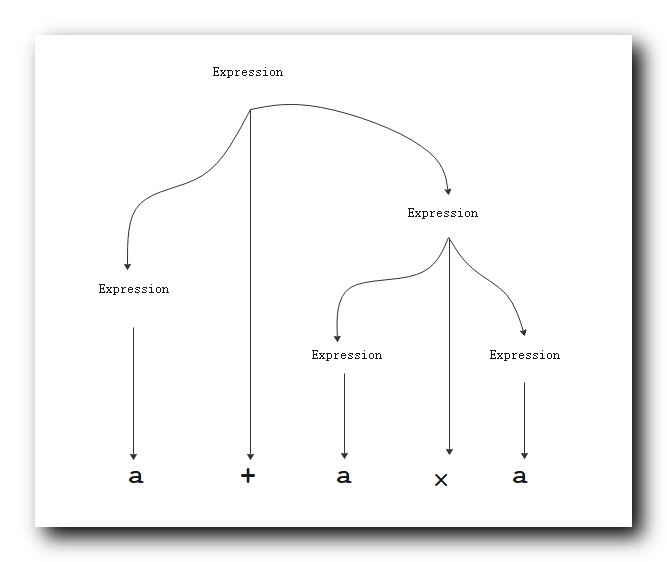2 . 在上述的 语法分析树中 , 加法优先级高于乘法 , 这是错误的分析 ;

② 语法分析树 2 :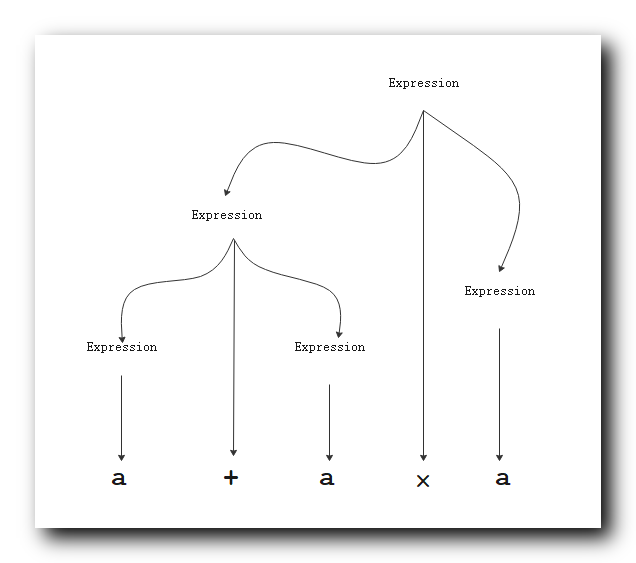在上述的 语法分析树中 , 乘法优先级高于加法 , 这是正确的分析 ;

3 . 语法歧义性分析 : 上述语法中是无法区分 加法 和 乘法的优先级的 , 因此这里得到两个完全不一致得我语法分析树 , 那么该语法是有歧义的 ;

4 . 与代数表达式语法对比 : 之前讲的代数表达式是好的语法 ,  乘法 和 加法的优先级 也体现出来 , 乘法优先级高于加法 , 括号的优先级高于乘法 ;

① 代数表达式语法 :

$Expression \to Expression + Term \quad | \quad Term$
$Term \to Term \times Factor \quad | \quad Factor$
$Factor \to Expression \quad | \quad a$

② 代数表达式语法分析树 : 这个语法分析树是唯一的 , 没有其它的形式 , 该语法是没有歧义的 ;③ 有歧义的语法 : 在本节的语法中 , 无法区分 加法 和 乘法的优先级 , 该语法是有歧义的 ;

5 . 总结 : 如果语法有歧义 , 那么中间的字符串有歧义 ; 没有算法 可以判定 上下文无关语法 是否有歧义 ; 有些语法天生就是有歧义的 , 但可以通过某种方法去掉语法中的歧义性 ;

三、Chomsky 范式

1 . Chomsky 范式 : 上下文无关语法中的任何规则都是如下格式 ;

① $A \to BC$ : $A$ 是 变元 , $B,C$ 也是变元 ;
② $A \to a$ : $A$ 是 变元 , $a$ 是常元 , $A$ 可以被终端字符替换 ;
③ $B ,C$ 变元要求 : $B, C$ 变元一定不能是开始变元 ;
④ $S \to \varepsilon$ : $S$ 开始变元可以为空 ;
⑤ 不能出现 $变元 \to 变元$ 单个变元 到 单个变元不允许出现 ;

2 . $S \to \varepsilon$ 规则 说明 :

① 语言包含空字符串 : 如果上下文无关语法包含空字符串时 , 一定需要 $S \to \varepsilon$ 规则 ;
② 语言不包含空字符串 : 如果上下文无关语法不包含空字符串时 , 一定不需要 $S \to \varepsilon$ 规则 ;
③ 规则总结 : 该规则决定 上下文无关语法 所生成的语言 是否包含 空字符串 , 如果包含必须要这个规则 , 如果不包含空字符串一定不要这个规则 ;

四、上下文无关语法 转为 Chomsky 范式

Chomsky 范式规则 的 上下文无关语法 生成的语言 的语法分析树 除叶子节点之外 都 是二叉树 , 叶子节点 与 上一层都是 一对一的节点 ;
任何 上下文无关语法 , 都可以找到一个 Chomsky 范式 与其等价 ;
任何 上下文无关语法 的语法分析树 都可以进行修剪 , 修剪后的树都是二叉树 ;

上下文无关语法 转为 Chomsky 范式 步骤 :

1 . 添加开始变元及规则 : 添加一个新的开始变元 $S_0$ , 以及配套的规则 $S_0 \to S$ , $S$ 是旧的开始变元 ;

① 目的 : 添加开始变元的目的是 开始变元 永远出现在左边 ;
② Chomsky 范式 中 , 开始变元始终在规则的左边 , 不允许开始变元在规则的右侧 ;
③ 对应 Chomsky 范式 规则 :  $A \to BC$ 规则 ,  $A$ 是 变元 , $B,C$ 也是变元 , 并且 $B,C$ 不允许是开始变元 ;

2 . 消除所有的 $\varepsilon$ 规则 : 消除所有从 变元 到 空字符 的规则 ;

3 . 消除所有的 $A \to B$ 规则 : 消除所有从 单个变元 到 单个变元的 单条规则 , 允许从 单个变元 到 多个变元或常元 ;
如 $A \to B$ 是需要删除的 , $A \to BS$ 可以保留 ;

五、上下文无关语法 转为 Chomsky 范式 示例

将 上下文无关语法 $G6$ 转为 Chomsky 范式 :

$S \to ASA | aB$
$A \to B|S$
$B \to b|\varepsilon$

转换过程如下 :

1 . 添加新的开始变元 : $S_0$ , 旧的开始变元 $S$ 就不是开始变元了 ;
当前的语法格式如下 :

$S_0 \to S$
$S \to ASA | aB$
$A \to B|S$
$B \to b|\varepsilon$

2 . 消除 $\varepsilon$ 规则 :

消除 $\varepsilon$ 规则 原则 : 假设有规则 $C \to \varepsilon$ , $D \to uCv$ , 如果要删除 $\varepsilon$ 规则 , 需要实现 消除前后具有 相同的替换效果 , 将规则改为 $D \to uv$ 即可删除 $\varepsilon$ 相关规则 ;
( 消除前后 , 替换效果必须一致 )

3 . 消除  $B \to b|\varepsilon$ 中的 $\varepsilon$ : 会影响 $S \to ASA | aB$ 和 $A \to B|S$ 两条规则中涉及到了 $B$ 变元 , 消除的原则是 " 消除前后 , 替换效果必须一致 " ;

3.1 .  $S \to ASA | aB$ 规则消除 $\varepsilon$ 分析 :   这里讨论 消除 $B \to b|\varepsilon$ 规则中的 $B \to \varepsilon$ 规则 对 $aB$ 的影响 ;

① 消除 $B \to \varepsilon$  规则前分析 : 使用 $B \to b|\varepsilon$ 规则 对 $aB$ 进行替换 有两种情况 , 分别是 $ab$ , $a$ , 两种情况 ;

② 消除 $B \to \varepsilon$  规则后分析 : 如果要消除 $B \to \varepsilon$ 规则 , 那么消除后的规则是 $B \to b$ , 使用 $B \to b$ 规则对 $aB$ 进行替换 , 其替换 结果必须是 $ab$ , $a$ , 两种情况 ;

分析 $ab$ , $a$ 两种结果 :

$aB$ 使用 $B \to b$ 规则替换 , 可以得到 $ab$ ;

$a$ 替换结果无法获取 , 此时需要在 $aB$ 的平级 , 再次添加 $a$ 即可达到上述效果 ;

$aB$ 最终修改方案 : 将 $aB$ 改为 $aB|a$ , 使用 $B \to b$ 规则替换 $aB|a$ 的结果是 $ab$ , $a$ , 与上述消除 $B \to \varepsilon$  规则 前的结果一致 ;

③ $S \to ASA | aB$ 规则对应的消除 $B \to \varepsilon$  规则后的结果为
$S \to ASA | aB | a$

④ 当前的语法格式如下 : 注意 还没有讨论 $A \to B|S$ 规则中的 $B$ , $B \to b|\varepsilon$ 规则中的 $\varepsilon$ 还不能删除 ;

$S_0 \to S$
$S \to ASA | aB | a$
$A \to B|S$ : 注意此时该规则不完善 , 还没有删除 $\varepsilon$ ;
$B \to b$

3.2 .  $A \to B|S$ 规则消除 $\varepsilon$ 分析 :   这里讨论 消除 $B \to b|\varepsilon$ 规则中的 $B \to \varepsilon$ 规则 对 $B$ 的影响 ;

① 消除 $B \to \varepsilon$  规则前分析 : 使用 $B \to b|\varepsilon$ 规则 对 $B$ 进行替换 有两种情况 , 分别是 $b$ , $\varepsilon$ , 两种情况 ;

② 消除 $B \to \varepsilon$  规则后分析 : 如果要消除 $B \to \varepsilon$ 规则 , 那么消除后的规则是 $B \to b$ , 使用 $B \to b$ 规则对 $B$ 进行替换 , 其替换 结果必须是 $b$ , $\varepsilon$ , 两种情况 ;

分析 $b$ , $\varepsilon$ 两种结果 :

$B$ 使用 $B \to b$ 规则替换 , 可以得到 $b$ ;

$\varepsilon$ 替换结果无法获取 , 此时需要在 $B$ 的平级 , 再次添加 $\varepsilon$ 即可达到上述效果 ;

$B$ 最终修改方案 : 将 $B$ 改为 $B|\varepsilon$ , 使用 $B \to b$ 规则替换 $B|\varepsilon$ 的结果是 $b$ , $\varepsilon$ , 与上述消除 $B \to \varepsilon$  规则 前的结果一致 ;

③ $A \to B|S$ 规则对应的消除 $B \to \varepsilon$  规则后的结果为
$A \to B| \varepsilon|S$

④ 当前的语法格式如下 : 注意 还没有讨论 $A \to B|S$ 规则中的 $B$ , $B \to b|\varepsilon$ 规则中的 $\varepsilon$ 还不能删除 ;

$S_0 \to S$
$S \to ASA | aB | a$
$A \to B| \varepsilon |S$
$B \to b$

4 . 消除  $A \to B| \varepsilon |S$ 中的 $\varepsilon$ : 会影响 $S \to ASA | aB | a$ 规则中涉及到了 $A$ 变元 , 消除的原则是 " 消除前后 , 替换效果必须一致 " ;

① 消除 $ASA$ 中的 $\varepsilon$ , 添加以下项即可 :

第一个 $A$ 通过 $\varepsilon$ 代替 : 添加 $SA$ 项 ;

第二个 $A$ 通过 $\varepsilon$ 代替 : 添加 $AS$ 项 ;

两个 $A$ 都通过 $\varepsilon$ 代替 : 是 $S$ , 可以不同写 , $S \to S$ 没啥意义 ;

② $S \to ASA | aB | a$ 规则对应的消除 $A \to \varepsilon$  规则后的结果为 :
$S \to ASA | AS | SA | aB | a$

③  当前的语法格式如下 :

$S_0 \to S$
$S \to ASA | AS | SA | aB | a$
$A \to B| S$
$B \to b$

5 . 消除 $A \to B$ 规则 :

假设要消除 $C \to D$ 规则 : 如果语法中有 $D \to W$ 规则 , 那么如果消除 $C \to D$ , 需要将 $C \to W$ 体现出来 ;

消除 $A \to B$ 规则 , 检查 $B$ 出现在规则左边的情况 , 这里有 $B \to b$ 规则 , 需要 添加 $A\to b$ 规则后 , 即可删除 $A \to B$ 规则 ;

删除前规则 :

$S_0 \to S$
$S \to ASA | AS | SA | aB | a$
$A \to B| S$
$B \to b$

删除后规则如下 :

$S_0 \to S$
$S \to ASA | AS | SA | aB | a$
$A \to b| S$

6 . 消除 $A \to S$ 规则 :

① 消除 $A \to S$ 规则 , 检查 $S$ 出现在规则左边的情况 , 这里有 $S \to ASA | AS | SA | aB | a$ 规则 , 需要 添加 $A\to ASA | AS | SA | aB | a$ 规则后 , 即可删除 $A \to S$ 规则 ;

② 删除前规则 :

$S_0 \to S$
$S \to ASA | AS | SA | aB | a$
$A \to b | S$

③ 删除后规则如下 :

$S_0 \to S$
$S \to ASA | AS | SA | aB | a$
$A \to b| ASA | AS | SA | aB | a$

7 . 分解规则 :

① 分解示例 : $S \to ASA$ 可以分解为 $S \to R$ , $R \to SA$
② 分解前的规则 :

$S_0 \to S$
$S \to ASA | AS | SA | aB | a$
$A \to b| ASA | AS | SA | aB | a$

③ 分解后的规则 :

$S_0 \to S$

下面的规则 是 $S \to ASA | AS | SA | aB | a$ 分解后的规则 :

$S \to R$
$R \to SA$
$S \to AS$
$S \to SA$
$S \to aB$
$S \to a$

下面的规则 是 $A \to b| ASA | AS | SA | aB | a$  分解后的规则 :

$A \to b$
$A \to R$
$A \to SA$
$A \to AS$
$A \to SA$
$A \to aB$
$A \to a$


展开全文计算理论
• 程序使用的语法上下文无关文法，是乔姆斯基分类法则中的第2类语法。 0 1 2 3 文法名称 非限制型文法 上下文有关文法 上下文无关文法 正则文法 对应机器 图灵机 线性（边界）...
分析可以用来确定程序的结构。程序使用的语法是上下文无关文法，是乔姆斯基分类法则中的第2类语法。

0
1
2
3

文法名称
非限制型文法
上下文有关文法
上下文无关文法
正则文法

对应机器
图灵机
线性（边界）自动机
下推自动机
有穷自动机

识别对象
自然语言
受限自然语言
程序语言
单词

上下文无关文法使用BNF文法表示。文法规则中有一个“推导”的行为，此行为在右边选一个结构名字替换序列。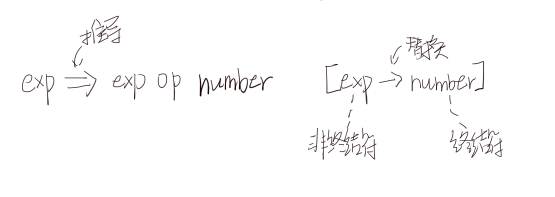推导中有最左推导和最右推导两种方法。
文法可能有二义性，可以通过两种方式来解决。一种是设立消除二义性规则；另一种是将文法变成一个强制正确分析书的构造的格式。
扩展的BNF即EBNF中有{…}和[…]两个符号。分别表示重复和可选。EBNF可以通过作图的方式展示出来。
上面提到，有两种方法可以去除文法的二义性。下面给出第二种解决二义性的方法的例题。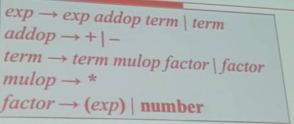上图中的强制文法通过左递归实现左结合，从而实现了结合性。
通过上面的文法来实现：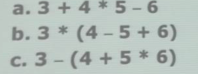a的最左推导为：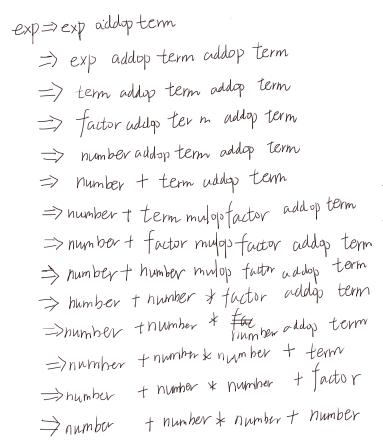a的分析树为：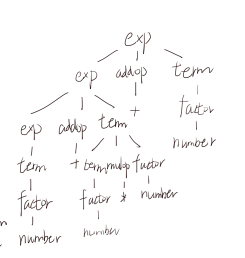a的抽象语法树为：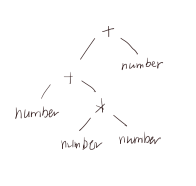b的分析树为：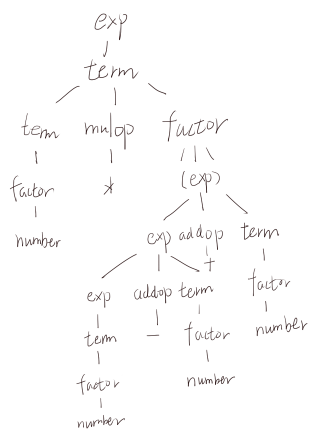b的抽象语法树为：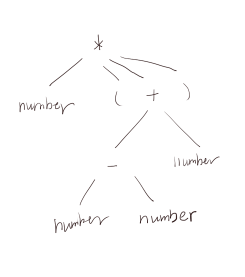c的分析树为：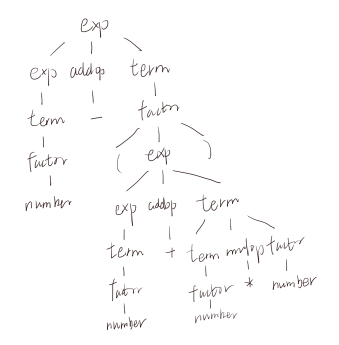c的抽象语法树为：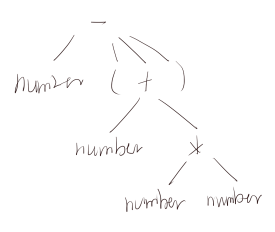参考资料：《编译原理与实践》


展开全文• ## 上下文无关文法

千次阅读 2019-10-08 11:21:59
本文的部分例子来自北京大学计算语言学...由形式语言理论提出，在计算语言学中广泛使用的“上下文无关文法”（Context Free Grammar，缩写为CFG，也被称作2型语法）并不是上下文无关的。之所以被称作“上下文无关...nlp
• 用到DFA有穷自动机的建立 构建识别活前缀的DFA利用项目集和状态转换函数建立LR(0)分析表 上下文无关文法测试 E->aA E->bB A->cA A->d B->cB B->d #
• ## 上下文无关语法

千次阅读 2018-01-06 21:34:31
本博文主要介绍基于巴科斯-诺尔范式的上下文无关语法，以及在计算机编程语言和解析技术上的应用。 引言 编程语言，协议规范，查询语言，文件格式，模式语言，内存布局，形式语言，配置文件，标记语言，格式化...解析
• 对于文法G=(V, T, S, P)，如果产生式的形式如下：A -> xBA -> x其中A, B属于V，x属于T*，则称为右线性文法；相似的，如果产生式的形式如下：A -> BxA -> x则称为左线性文法。右线性文法和左线性文法统称...
• 1型文法上下文有关文法。（任何产生规则的左手端和右手端都可以被终结符和非终结符的上下文所围绕，乔姆斯基描述自然语言的一种方式介入的，在自然语言中一个单词是否可以出现在特定的位置要依赖于上下文。） ...
• 正规的语法特点 1.全部长度有限的语言都是正规的。 2.用正规文法当然能产生无限长串，当中周期反复部分的长度不大于非终止符的长度。...剩下的那部分我们叫做真正的上下文无关文法。 自嵌入特性是...
• 语法规则：上下文无关文法（子集－LL文法或LR文法） 语法分析：下推自动机（LL或LR分析器），自上而下和自下而上分析 （这两种都只能处理上下文无关文法的子类） 语法分析器 语法分析器是编译器前端的重要组成部分...编译原理
•编译原理 词法分析
• 上下文无关文法是程序设计语言所使用的语法。它的特点是同样的字符串在不同的语境下，意思不变。满足上下文无关文法的语言便于计算机识别和处理。我们已经介绍过，语言是语句的集合，而语句是通过产生式定义的。...
• 正则表达式 上下文无关by Christopher Diggins... 正则表达式之外：解析上下文无关语法的简介 (Beyond regular expressions: An introduction to parsing context-free grammars) An important and useful tool that...编程语言 python java 大数据 人工智能
• 上下文无关文法及分析3.1 分析过程3.2 上下文无关文法3.3 分析树与抽象语法树3.4 二义性3.5 扩展的表示法：EBNF和语法图3.6 上下文无关语言的形式特性3.7 TINY语言的语法 分析的任务是确定程序的语法，或称作结构，...编译器 编程语言
• 上下文无关文法判定的动态规划实现程序，按照ACM编程提交方案编写main函数。
• 上下文无关文法的表示与存储（Java描述） 【问题描述】 把输入的文法存储在计算机内。 【基本要求】 1、输入上下文无关文法的一组产生式。 2、将文法按顺序或链式结构存储在计算机内。 3、输出文法的四要素：终极符...编译原理 实验 Java
• 在龙书Compilers - Principles, Techniques, & Tools英文版第2版42页中，提到上下文无关文法有以下的特点： 一个终结符的有限集（A set of terminal symbols），构成文法的最基本的字符就是这个文法的终结符，例如...编译原理
• 一、乔姆斯基范式、 二、上下文无关语法转为乔姆斯基范式步骤、 三、上下文无关语法转为乔姆斯基范式示例1、 四、上下文无关语法转为乔姆斯基范式示例 2计算理论 乔姆斯基范式
• 在龙书Compilers - Principles, Techniques, & Tools英文版第2版42页中，提到上下文无关文法有以下的特点：  一个终结符的有限集（A set of terminal symbols），构成文法的最基本的字符就是这个文法的终结符，...NLP
• 最近在学习编译原理相关知识，主要看的是编译器前端分析技术，主要学习的有词法分析、语法分析、语义分析、有限自动机、上下文无关文法、BNF范式、语法分析树等相关前端概念内容，后续可能使用Anltr或Peg对特定的DSL...编译原理 有限自动机 词法分析
• 文章目录知识点重难点上下文无关文法的简化无用符号杂项 知识点 推导 最左推导（只变换最左边的非终结符）与最右推导 归约 n步推导符号：* 推导树（语法树，语法分析树） 根：文法的起始非终结符 非叶子结点：...
• Part 1 短语结构文法（PSG）、上下文有关文法（CSG）、上下文无关文法（CFG）、右线性文法(RLG)的区别 Part 2 概念 文法G=(V，T，P，S) G叫做0型文法(type 0 grammar)，也叫做短语结构文法(phrase structure ...有限自动机
• 本文是5分钟理解CFG上下文无关文法的续集，在5分钟理解CFG上下文无关文法这篇文章中已经讲解了CFG的基本概念，但是CFG的解析算法才是核心。由于它的解析算法极其复杂，网上很少有文章能把解析算法用大众能理解的语言...自然语言处理 nlp
• 该章主要内容： 上下文无关文法（CFG） Chomsky范式和，Greibach...上下文无关文法对应的识别器是下推自动机。 确定的下推自动机对应于上下文无关语言的一个子集（大部分的程序设计语言）。 3.1 推导树与二义性 ......